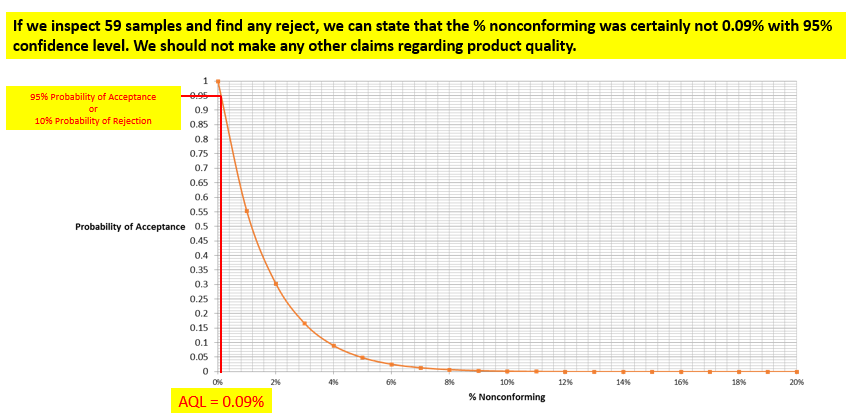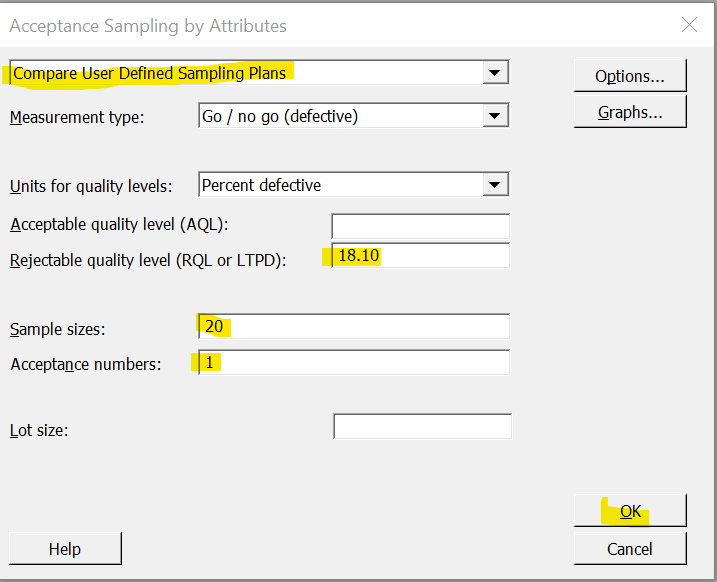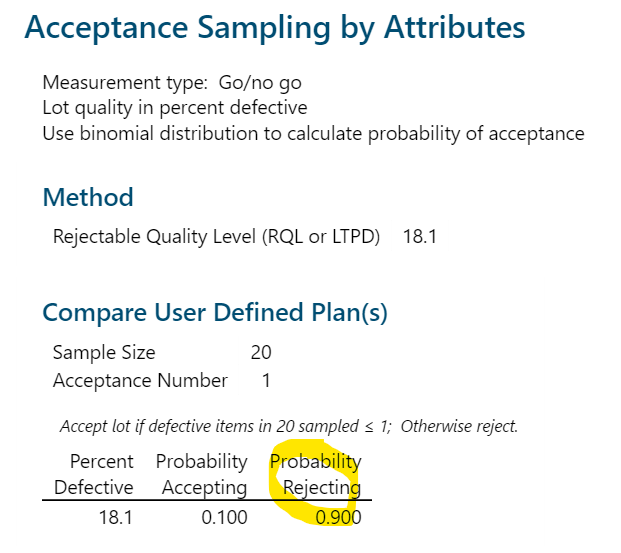#Quality Digest

Featured Product
This Week in Quality Digest Live
Management Features
Jake Mazulewicz
Three tips from high-reliability organizations
Dave Gilson
Getting out of the boardroom for a stroll changes how women navigate
Bob Ferrone
Saving the planet and bolstering the bottom line
Jeanne Quimby
Kids can be the source of new ideas
Matt Fieldman
Vocational programs and apprenticeships show the way
Management News
How to drive productivity with a universal and powerful 3D inspection software
Research commissioned by the Aerospace & Defense PLM Action Group with Eurostep and leading PLM providers
Remote Not Distant
Improved design of polarization-independent beam splitters
New industry-recognized guidelines for manufacturing jobs
ASQ will address absence of internationally recognized ESG benchmarks
Helping organizations improve quality and performance
Leading technologies empowering the next generation of 3D engineering software solutions
EstateSpace offers digital estate management systemBio

Management

## OC Curve and Reliability/Confidence Sample Sizes

### Using OC curves to generate reliability/confidence values

Published: Wednesday, May 24, 2023 - 12:03

I’m looking at a topic in statistics. I’ve had a lot of feedback on one of my earlier posts on OC curves and how one can use them to generate a reliability/confidence statement based on sample size (n), and rejects (c). I provided an Excel spreadsheet that calculates the reliability/confidence based on sample size and rejects. I’ve been asked how we can use Minitab to generate the same results. So, this post is mostly geared to using OC curves to generate reliability/confidence values and using Minitab to do the same.

The basic premise is that a Type B OC curve can be drawn for samples tested, n and rejects found, c. On the OC curve, the line represents various combinations of reliability and confidence. The OC curve is a plot between percent nonconforming and probability of acceptance. The lower the percent nonconforming, the higher the probability of acceptance. The probability can be calculated using binomial, hypergeometric, or Poisson distributions. The binomial OC curves are called “Type B” OC curves and don’t use lot sizes, generally represented as N. The hypergeometric OC curves do use lot sizes and are called “Type A” OC curves. When the ratio n/N is small and n >= 15, the binomial distribution closely matches the hypergeometric distribution. Therefore, the Type B OC curve is used quite often.

The most commonly used standard for attribute sample plans is MIL 105E. The sample plans in MIL 105E are identical to the Z1.4 standard plans. The sampling plans provided as part of the tables do use lot sizes. These sampling plans were “tweaked” to include lot sizes because there was a push for including economic considerations of accepting a large lot that might contain rejects. The sample sizes for larger lots were made larger due to this. The OC curves shown in the standards, however, are Type B OC curves that do not use lot sizes. Hypergeometric distribution considers the fact that there’s no replacement for the samples tested. Each test sample removed will affect the subsequent testing because the number of samples is now less. However, as noted above, when the ratio n/N is small, the issue of not replacing samples isn’t a concern. For the binomial distribution, lot size isn’t considered because the samples are assumed to be taken from lots of infinite lot size.

With this background, let’s look at a Type B OC curve. The OC curve is a plot between percent nonconforming and probability of acceptance. The lower the percent nonconforming, the higher the probability of acceptance. The OC curve shown is for n = 59 with 0 rejects calculated using binomial distribution.The producer’s risk is the risk of good product being rejected. The acceptance quality limit (AQL) is generally defined as the percent of defects that the plan will accept 95 percent of the time (i.e., in the long run). Lots that are at or better than the AQL will be accepted 95 percent of the time (in the long run). If the lot fails, we can say with 95-percent confidence that the lot quality level is worse than the AQL. Likewise, we can say that a lot at the AQL that is acceptable has a 5-percent chance of being rejected. In the example, the AQL is 0.09 percent.The consumer’s risk, on the other hand, is the risk of accepting a bad product. The lot tolerance percent defective (LTPD) is generally defined as the percent of defective product that the plan will reject 90 percent of the time (in the long run). We can say that a lot at or worse than the LTPD will be rejected 90 percent of the time (in the long run). If the lot passes, we can say with 90-percent confidence that the lot quality is better than the LTPD (i.e., the percent nonconforming is less than the LTPD value). We could also say that a lot at the LTPD that is defective has a 10-percent chance of being accepted.

The vertical axis (y axis) of the OC curve goes from 0 percent to 100 percent probability of acceptance. Alternatively, we can say that the y axis corresponds to 100 percent to 0 percent probability of rejection. Let’s call this confidence. This is also the probability of rejecting the lot. The horizontal axis (x axis) of the OC curve goes from 0 percent to 100 percent for percent nonconforming. Alternatively, we can say that the x axis corresponds to 100 percent to 0 percent for percent conforming. Let’s call this reliability.We can easily invert the y axis so it aligns with a 0 to 100-percent confidence level. In addition, we can also invert the x axis so it aligns with a 0 to 100-percent reliability level. This is shown below.The OC curve line is a combination of reliability and confidence values. Therefore, for any sample size and rejects combination, we can find the required combination of reliability and confidence values. If we know the sample size and rejects, then we can find the confidence value for any reliability value or vice versa. Let’s look at a problem to detail this further:

In the wonderful book Acceptance Sampling in Quality Control, by Edward Schilling and Dean Neubauer (Chapman and Hall/CRC, 2009 second edition), the authors discuss a problem that would be of interest here. They posed:

“...consider an example given by Mann et al. rephrased as follows: Suppose that n = 20, and the observed number of failures is x = 1. What is the reliability π of the units sampled with 90-percent confidence? Here, π is unknown and γ is to be .90.”

One of the solutions given was to find the reliability or the confidence desired directly from the OC curve.

They gave the following relation:

π = 1 – p, where π is the reliability and p is the nonconforming rate

γ = 1 – Pa, where γ is the confidence and Pa is the probability of acceptance

This is the same relation that was explained above.

In my spreadsheet, when we enter the values as shown below, we see that the reliability value is 81.91 percent based on LTPD value of 18.10 percent. This is the same result documented in the book.We can use Minitab to get the same result. However, it will be slightly backward. As I noted above, drawing the OC curve requires only two inputs: the sample size and the number of rejects allowed (or acceptance number). Once the OC curve is drawn, we can then look at the different reliability and confidence combinations. We can also calculate the confidence if we provide the reliability. The reliability is also 1 – p. In Minitab, we can input the sample size, number of rejects, and p, and the software will give us the Pa. For the purpose of reliability and confidence, the p value will be the LTPD value, and the confidence value will be 1 – Pa.

I’m using Minitab 18 here. Go to Acceptance Sampling by Attributes as shown below:Choose “Compare User Defined Sampling Plans” from the dropdown and enter the different values as shown. Please note that the acceptance number is the maximum number of rejects allowed. Here we’re entering the LTPD value because we know the value to be 18.10. In the spreadsheet, we have to enter the confidence level we want to calculate the reliability, while in Minitab we have to enter the LTPD value (1 – reliability) to calculate the confidence. In the example below, we’re going to show that entering the LTPD as 18.10 will yield the Pa as 0.10, and thus the confidence as 0.90, or 90 percent.Minitab yields the following result:One can use the combination of sample size, acceptance number, and required LTPD value to calculate the confidence value. The spreadsheet is available here.

I’ll finish with one of the oldest statistical quotes, written by the 16th-century Spanish author of Don Quixote, Miguel de Cervantes: “The proof of the pudding is in the eating.” Earlier in the same book, he wrote, “By a small sample we may judge of the whole piece.”